# Zero-one law

(diff) ← Older revision | Latest revision (diff) | Newer revision → (diff)

The statement in probability theory that every event (a so-called tail event) whose occurrence is determined by arbitrarily distant elements of a sequence of independent random events or random variables has probabilityor. This law extends to systems of random variables depending on a continuous parameter (see below).

For individual tail events the fact that their probability isorwas established at the beginning of the 20th century. Thus, let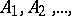be a sequence of independent events. Letbe the tail event that infinitely many events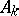occur, i.e.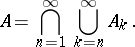Then, as noted by E. Borel , eitherBy a simple calculation he showed thatand(see Borel–Cantelli lemma).

Next, if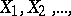is a sequence of independent random variables, then the probability that the series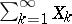converges can only beor. This fact (together with a criterion that makes it possible to distinguish these two cases) was established by A.N. Kolmogorov in 1928 (see , ).

Tail events connected with analytic properties of sums of sequences of functions, for example, power series with random terms, have also been investigated. Thus, Borel's vague assertion (1896) that for "arbitrary coefficients" the boundary of the disc of convergence is the natural boundary of the analytic function represented by the coefficients was put in the following precise form by H. Steinhaus . Letbe independent random variables uniformly distributed on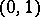(cf. Uniform distribution), let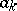be given numbers and suppose that the power series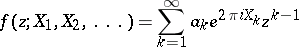has radius of convergence. Then the (tail) event that the functioncannot be extended across the boundary of the disc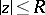has probability. B. Jessen  has proved that any tail event connected with a sequence of independent random variables that are uniformly distributed onhas probabilityor.

A general zero-one law was stated by Kolmogorov (see ) as follows. Letbe a sequence of random variables and let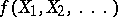be a Borel-measurable function such that the conditional probability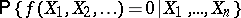of the relation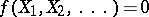given the firstvariablesis equal to the unconditional probability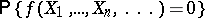(*)

for every. Under these conditions the probability (*) isor. For independentthe zero-one law as stated at the beginning of the article follows from this.

As P. Lévy proved in 1937 (see ), Kolmogorov's theorem follows from a more general property of conditional probabilities, namely that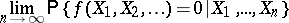almost certainly equalsor(depending on whetheris zero or not). In turn, this assertion follows from a theorem on martingales (see , Chapt. III, Sect. 1; Chapt. VII, Sects. 4, 5, 7 and the comments; in Sect. 11 there is an analogue of the zero-one law for random processes with independent increments; this implies, in particular, that sample distribution functions of a separable Gaussian process with continuous correlation function are continuous with probabilityat every point or have, with probability, a discontinuity of the second kind at every point; see also ).

For the special case of a sequenceof independent and identically-distributed random variables it has been shown (see ) that the probability not only of any tail event, but also of any event that is invariant under any permutation of finitely many terms of the sequence isor.

How to Cite This Entry:
Zero-one law. Encyclopedia of Mathematics. URL: http://encyclopediaofmath.org/index.php?title=Zero-one_law&oldid=11252
This article was adapted from an original article by A.V. ProkhorovYu.V. Prokhorov (originator), which appeared in Encyclopedia of Mathematics - ISBN 1402006098. See original article